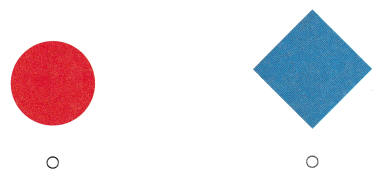# Texas Go Math Kindergarten Lesson 17.4 Answer Key Identify Squares

Refer to our Texas Go Math Kindergarten Answer Key Pdf to score good marks in the exams. Test yourself by practicing the problems from Texas Go Math Kindergarten Lesson 17.4 Answer Key Identify Squares.

## Texas Go Math Kindergarten Lesson 17.4 Answer Key Identify Squares

Essential Question
How can you identify, sort, and describe squares?

Explore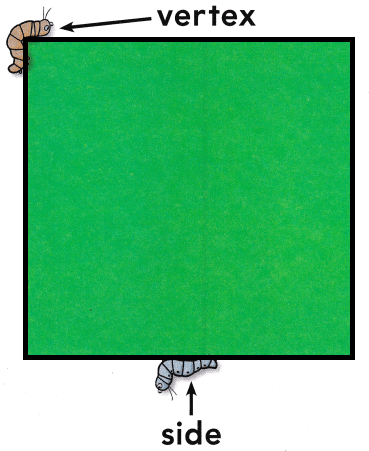Explanation:
A square has 4 sides and 4 vertices
all the four sides are equal
opposite sides are parallel

Directions
Use your finger to trace around the square. Talk about the number of sides and the number of square vertices, Draw an arrow pointing to another square vertex. Use a pencil or crayon to trace around the square.

Share and Show

Question 1.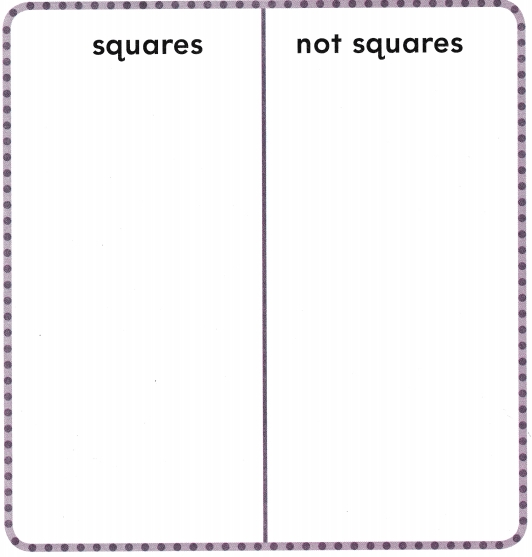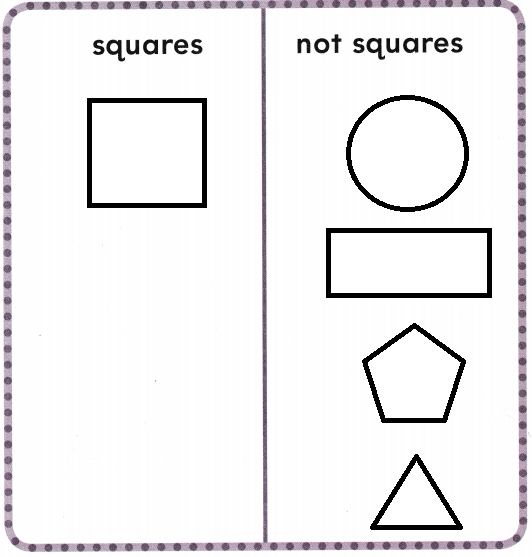Explanation:
A square has 4 sides and 4 vertices
all the four sides are equal
opposite sides are parallel

Directions
1. Use two-dimensional shapes. Sort the shapes by squares and not squares. Trace and color the shapes on the sorting mat.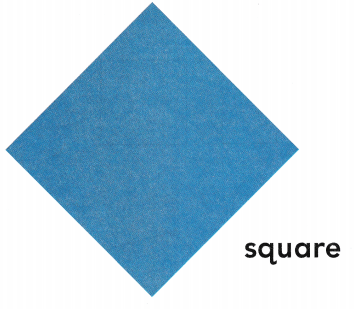Question 2.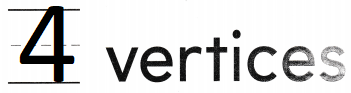Explanation:
A square has 4 sides and 4 vertices
all the four sides are equal
opposite sides are parallel

Question 3.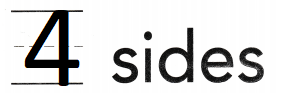Explanation:
A square has 4 sides and 4 vertices
all the four sides are equal
opposite sides are parallel

Directions
2. Place a counter on each corner, or vertex. Write how many corners, or vertices. 3. Trace around the sides. Write how many sides.

Home Activity

• Have your child show you an object that is shaped like a square.

Problem Solving

Question 4.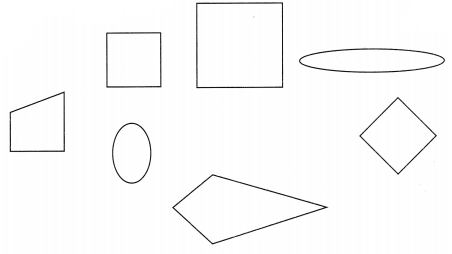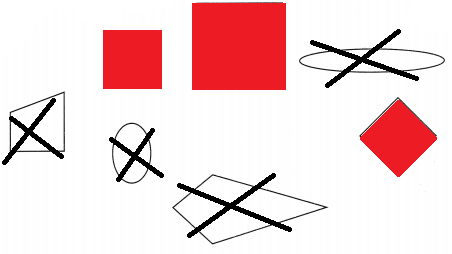Explanation:
A square has 4 sides and 4 vertices
all the four sides are equal
opposite sides are parallel
Colored with red for all the squares
crossed all the shapes that are not squares

Question 5.Explanation:
A square has 4 sides and 4 vertices
all the four sides are equal
opposite sides are parallel
The first shape is a square
and the second shape is a rectangle
so, marked the first shape.

Directions
4. Sort the shapes. Use red to color the shapes that are squares. Mark on X on the shapes that are not squares. 5. Choose the correct answer. Which shape has four sides of equal length and four square vertices?

### Texas Go Math Kindergarten Lesson 17.4 Homework and Practice

Question 1.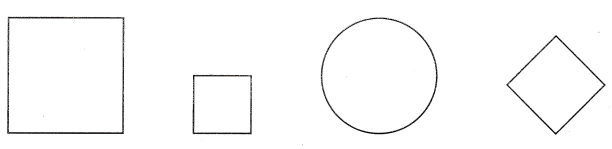Explanation:
A square has 4 sides and 4 vertices
all the four sides are equal
opposite sides are parallel

Question 2.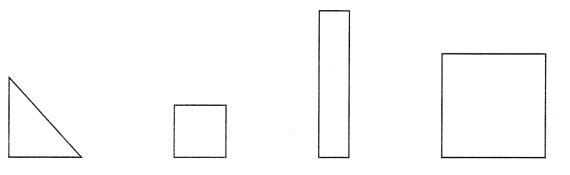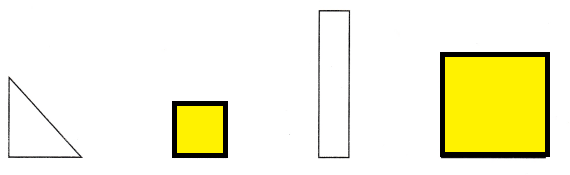Explanation:
A square has 4 sides and 4 vertices
all the four sides are equal
opposite sides are parallel

Question 3.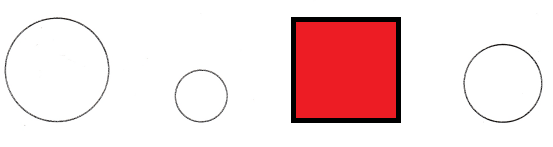Explanation:
A square has 4 sides and 4 vertices
all the four sides are equal
opposite sides are parallel

Directions
1-2. Identify and name the squares. Color the squares. 3. Identify and name the shapes that are not squares. Color those shapes.

Texas Test Prep

Lesson Check

Question 4.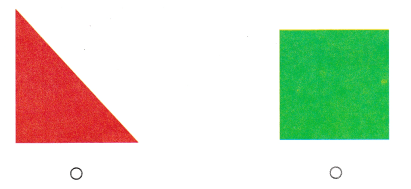Explanation:
A square has 4 sides and 4 vertices
all the four sides are equal
opposite sides are parallel

Question 5.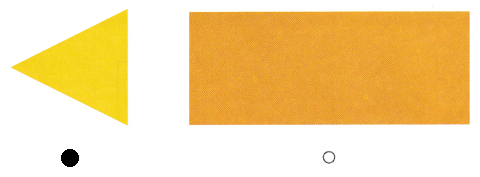Explanation:
A triangle has 3 sides and 3 vertices
so, marked the triangle

Question 6.